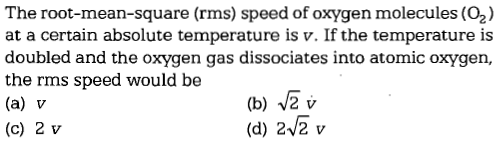• Subject:

...

• Topic:

...The root-mean-square (rms) speed of oxygenmolecules $\left({O}_{2}\right)$ at a certain absolute temperature is v. If the temperature is doubled and the oxygen gas dissociates into atomic oxygen, the rms speed would be

(a) V                        (b) $\sqrt{2}v$

(c) 2 v                     (d) 2 $\sqrt{2}v$